# What is new with Koide sum rules?

• arivero
Piotr Zenczykowski has appeared in this thread before (#74, #93). Today he points out that the modified gravitational law of "MOND" can be expressed in terms of the square root of mass, something which also turns up in Koide's formula.

•ohwilleke
The notion of expressing it in terms of the square root of mass has merit. I must say, however, that I find the inclination of the linked paper to frame the discussion in terms of classical Greek natural philosophers a real blow to the credibility of the overall presentation.

does not mention Koide but just cubic equations... using the cosine form. Not sure if it is a known trick in algebra.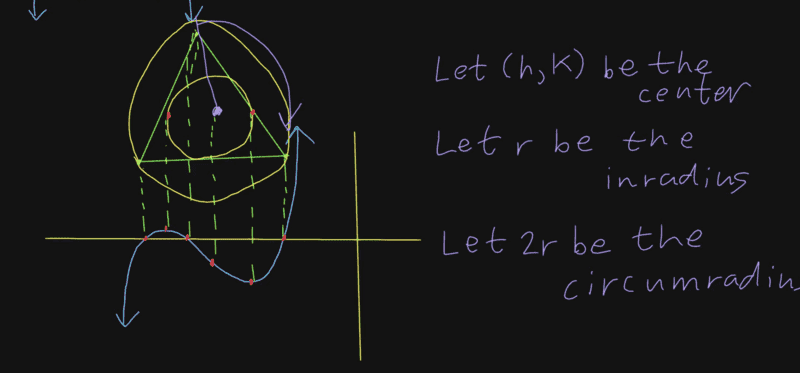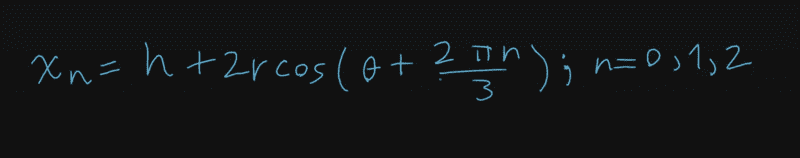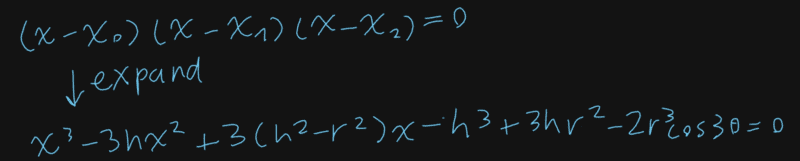You can check this in wolfram alpha,:
https://www.wolframalpha.com/input/?i=(x+-+h+-+2+r+cos+t)(x+-+h+-+2+r+cos+(t+++2+pi/3))(x+-+h+-+2+r+cos+(t+++4+pi/3))

Check the last minutes for more thoughts on the relation between cubic equation, conformal maps and Martin (Morgan? Moivre?) theorem.

The idea of using roots of cubic equation was mentioned before,

and indeed the equation

$$(\nu - x) (\nu^2 + 4 \nu x - 2 x^2) - \nu^3 \sqrt{2} \cos(3 \delta) = 0$$

meets, when expanded, the requirement b^2=6ac of the previous post, but note that the independent term

$$\nu^3 (1 - \sqrt{2} \cos(3 \delta) )$$

is not completely free; of course it is subject to the requirement of producing three real solutions. It is interesting here to note that the tuples for 15 and 45 degrees cancel the sqrt(2).

Other forms:

$$2 \left({x \over \nu} -1\right) \left({x \over \nu} - (1+\sqrt{3\over 2} )\right) \left( {x \over \nu} - (1-\sqrt{3\over 2})\right) = \sqrt{2} \cos(3 \delta)$$

$$\left({x \over \nu} -1\right) \left({x \over \nu} - (1+\sqrt{3\over 2} )\right) \left( {x \over \nu} - (1-\sqrt{3\over 2})\right) = \cos(\pi/4) \cos(3 \delta)$$

$$\left({x \over \nu} -1\right) \left({x \over \nu} - (1+\sqrt{3\over 2} )\right) \left( {x \over \nu} - (1-\sqrt{3\over 2})\right) = \frac 12 (\cos(3 \delta + \frac{\pi}{4} ) + \cos(3 \delta - \frac{\pi}{4} ))$$

EDIT: of course, a way via characteristic polynomials of matrices allow very easily to find formulae for mass alone, without roots, or for mass square. Not sure how the result would be different of Goffinet's https://www.physicsforums.com/threads/what-is-new-with-koide-sum-rules.551549/post-4269684 or of the original derivation of the formula.

EDIT2:
for a general cubic equation,
$$a x^3 + b x^2 + c x^2 + d = 0$$
if the three roots are real, then
$${ x_1^2 + x_2^2 + x_3^2 \over (x_1+x_2+x_3)} = 1 - {2 a c \over b^2}$$

EDIT3:

I am pondering if the expanded equation

$$2 x^3 - 6 \nu x^2 + 3 \nu^2 x + \nu^3 ( 1+ \sqrt{2} \cos(3 \delta) ) = 0$$

Could be seen as a condition for extremal (both maximum and minimum) of

$$\frac {1}{2} x^4 - 2 \nu x^3 + \frac{3}{2} \nu^2 x^2 + \nu^3 ( 1+ \sqrt{2} \cos(3 \delta) ) x$$

Very far fetched, but the coefficients are simple.

Last edited:
My intuition (guess) on the square root in the Koide equation is that it is in the nature of waves that their energies (and therefore their masses) are proportional to the squares of their amplitudes. Here "amplitude" is something about a wave that is convenient for mathematical physicists in that it is linear. Amplitudes are squared to get probabilities and it is probabilities that are proportional to energies and masses. We would do all our calculations in the probability / energy / mass units instead of amplitudes except that we would lose the convenient linearity. So it's natural to use square roots of mass when we're looking for linear equations relating mass / probability wave functions.

Now I also prefer density matrices to state vectors and this is the same relationship. In my view state vectors are not a part of reality, it is the density matrices that are fundamental. The state vectors are just a convenient way of making things linear so that we can use linear algebra to do calculations. But if I want a linear relationship between stuff represented by density matrices it is again natural to think about square roots.

And my paper on the subject of density matrix symmetry (which is a generalization of state vector symmetry) and the Standard Model is still "reviewers assigned" at Foundations of Physics now since May 2021.

***** Now for some fairly incoherent speculations *******

For the square root argument about MOND, I speculated back in 2003 that MOND might be related to the quantum Zeno effect. That effect is basically about the inability of a state vector to give exponential decay in the weak limit. The reason has to do with the square root relationship between the probability and the amplitude. Here's a link:
http://brannenworks.com/PenGrav.html

That references a 2003 write-up of mine titled "Ether, Relativity, Gauges and Quantum Mechanics" which is rather out of date. What I agree with it now is that position is discrete, that is, spatially the universe is a cubic lattice and time is also discrete. Maybe this means that velocity is quantized; that is, there is a minimum velocity. It's some tiny fraction of the speed of light.

•ohwilleke
I wonder if given the degree 3 equation, could it be useful to work out some mass matrices. For instance the matrix

$$M^\frac{1}{2}= \nu \begin{pmatrix} 1 & 1 & 0 \\ 0 & 1 +\sqrt \frac 32 & {\sqrt 2 \over 2} \cos (3\delta) \\ 1 & 0 & 1 - \sqrt \frac 32 \end{pmatrix}$$

should have eigenvalues meeting Koide formula. With a symmetric matrix, we could square it to produce other equivalent formulae. I am wondering if one needs to ask for normality of $M^\frac{1}{2}$, symmetry, or some other property, in order to get a valid mass matrix.

At the end of the day, Koide formula is about the quadratic invariant of a matrix, or the quotient between the quadratic invariant and the linear invariant.

Last edited:
•ohwilleke
Has someone reviewed this one?

https://arxiv.org/abs/2108.05787 Majorana Neutrinos, Exceptional Jordan Algebra, and Mass Ratios for Charged Fermions by Vivan Bhatt, Rajrupa Mondal, Vatsalya Vaibhav, Tejinder P. Singh

It is obscure, not easy read. But it uses, or finds, the polynomial equation form of Koide formula. Acording a blog entry, they will at some time ship a v2 with enhanced readability. Meanwhile T.P. Singh seems to update versions of a similar paper faster here, and has a previous blog post here about it.

Last edited:
•CarlB and ohwilleke
They don't aim to produce the Koide formula specifically. They just try to match mass ratios using combinations of eigenvalues of various matrices, and then use the Koide formula as an extra check.

The formulas for electron, muon, tau ratios are equations 56, 57 (an alternative that doesn't work as well is in equations 62, 63). The numbers they combine to create these formulas, are found in figure 1, page 21. They have no explanation for the particular combinations they use (page 23: "a deeper understanding... remains to be found"; page 25: "further work is in progress").

•arivero and ohwilleke
•ohwilleke and CarlB
One of the issues that has been raised about Koide's rule is that masses run with energy scale, unless you interpret it as applying only to pole masses.

It has also been noted that Koide's rule (and extensions of it) are really just functions of the mass ratios of particles and not their absolute masses.

With that in mind, some observations about the running of masses and mass ratios at high energies in the Standard Model in a recent preprint bear mentioning:
The CKM elements run due to the fact that the Yukawa couplings run. Furthermore, the running of the CKM matrix is related to the fact that the running of the Yukawa couplings is not universal. If all the Yukawa couplings ran in the same way, the matrices that diagonalize them would not run. Thus, it is the nonuniversality of the Yukawa coupling running that results in CKM running.
Since only the Yukawa coupling of the top quark is large, that is, O(1), to a good approximation we can neglect all the other Yukawa couplings. There are three consequences of this approximation:
1. The CKM matrix elements do not run below m(t).
2. The quark mass ratios are constant except for those that involve m(t).
3. The only Wolfenstein parameter that runs is A.
The first two results above are easy to understand, while the third one requires some explanation. A is the parameter that appears in the mixing of the third generation with the first two generations, and thus is sensitive to the running of the top Yukawa coupling. λ mainly encodes 1–2 mixing — that is, between the first and second generations — and is therefore insensitive to the top quark. The last two parameters, η and ρ, separate the 1–3 and 2–3 mixing. Thus they are effectively just a 1–2 mixing on top of the 2–3 mixing that is generated by A. We see that, to a good approximation, it is only A that connects the third generation to the first and second, and thus it is the only one that runs.
The preprint is Yuval Grossman, Ameen Ismail, Joshua T. Ruderman, Tien-Hsueh Tsai, "CKM substructure from the weak to the Planck scale" arXiv:2201.10561 (January 25, 2022).

The preprint also identifies 19 notable relationships between the elements of the CKM matrix at particular energy scales with one in particular that is singled out.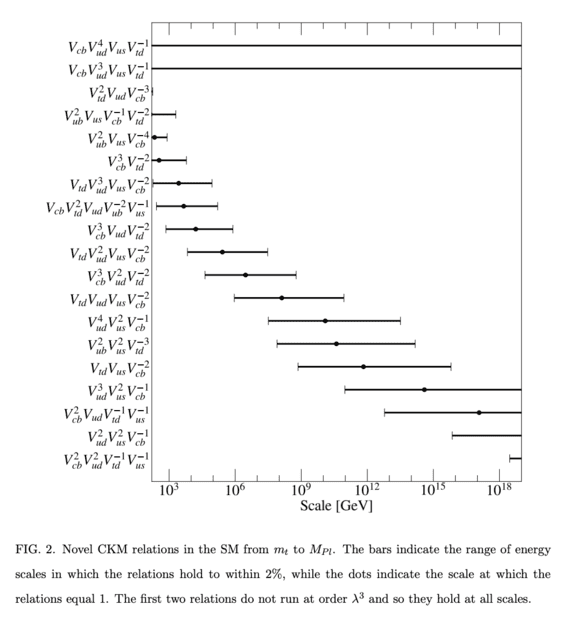At low energies, A2 which is the factor by which the probability associated with the CKM matrix entries that it is present in is consistent with being exactly 2/3rds.

The parameter "A" grows by 13% from the weak energy scale to the Planck energy scale, which means that A2 is about 0.846 at the Planck energy scale (about 11/13ths).

FWIW, I'm not convinced that it is appropriate to just ignore the running of the other Wolfenstein parameters, however, since if A increases, then one or more of the other parameters need to compensate downward, at least a bit, to preserve the unitarity of the probabilities implied by the CKM matrix which is one of its theoretically important attributes.

For convenient reference, the Wolfenstein parameterization is as follows: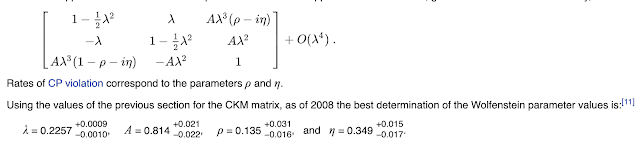Last edited:
If we take Koide's observation that his equation is perfect only at low energy limit, it calls into question the usual scheme of trying to understand the Standard Model as the result of symmetry breaking from something that is simplest at the high temperature limit.

Contrary to that symmetry breaking assumption, the history of 100 years work on the Standard Model is that as energies increase, our model becomes more complicated, not simpler. Maybe Alexander Unzicker is right and we're actually abusing symmetry instead of using it. As you generalize from symmetries to broken symmetries you increase the number of parameters in what is essentially a curve fitting exercise. People complain about the 10 ^ 500 models in string theory but the number of possible symmetries is also huge, given our ability to choose among an infinite number of symmetries each with an infinite number of representations.

My paper which shows that the mixed density matrices have more general symmetries seems to have finally reached the "under review" status at Foundations of Physics. It had been "reviewers assigned" since I sent it in in May last year, other than a few days but it went to "under review" on January 17 and is still there. I suppose they've got reviews back and are arguing about it. That paper's solution to the symmetry problem is to use mixed density matrices which can cover situations where the symmetry depends on temperature which is just what is needed for the Standard Model. But density matrices are incompatible with a quantum vacuum; instead of creation and annihilation operators you'd have to use "interaction operators" where, for example, an up-quark is created, a down quark annihilated and simultaneously a W- is created. But you couldn't split these up into the individual operators for the same reason you don't split density matrices into two state vectors (from the density matrix point of view).

•ohwilleke and arivero
Indeed SO(32) is huge if interpreted in the usual way, but I was surprised that the idea of the sBootstrap implies very naturally SO(15)xSO(15)

As for the low energy limit... I wonder if it relates to QCD mass gap. We have the numerical coincidence of 313 MeV

(EDIT: https://arxiv.org/abs/2201.09747 calculates 312 (27) MeV, but it compares with the lattice estimate 353.6 (1.1) and with longitudinal Schwinger with gives 320 (35). And then we have the issue of renormalization scheme )

Last edited:
•ohwilleke
density matrices are incompatible with a quantum vacuum; instead of creation and annihilation operators you'd have to use "interaction operators" where, for example, an up-quark is created, a down quark annihilated and simultaneously a W- is created. But you couldn't split these up into the individual operators for the same reason you don't split density matrices into two state vectors (from the density matrix point of view).
Is this formalism of nonfactorizable thermal interaction terms already described somewhere? Or do we have to wait for the paper?

•CarlB
Zenczykowski has written a follow-up to the MOND/Koide paper mentioned in #246, "Modified Newtonian dynamics and excited hadrons". This one does not mention Koide relations at all, so it's getting a little off-topic, but so we can understand his paradigm, I'll summarize it.

In hadronic physics, there is a "missing resonance" problem. The ground states of multiquark combinations are there as predicted by QCD, but there are fewer excited states than e.g. a three-quark model of baryons would predict. The conventional explanation of this seems to be, diquarks: quarks correlate in pairs and so in effect there are only two degrees of freedom (quark and diquark) rather than three, and therefore there are fewer possible states. (As another explanation, I will also mention Tamar Friedmann's papers, "No radial excitations in low energy QCD", I and II, which propose that radial excitations of hadrons don't exist,, and that they shrink rather than expand when you add energy.)

Zenczykowski's idea is that space is partly emergent on hadronic scales, e.g. that there are only two spatial dimensions there, and that this is the reason for the fewer degrees of freedom. This is reminiscent of the "infinite momentum frame", various pancake models of a relativistically flattened proton, and even the idea of a space-time uncertainty relation (in addition to the usual position/momentum uncertainty) that often shows up in quantum gravity.

The weird thing he does in the current paper, is to draw a line on a log-log graph of mass vs radius, indicating where the Newtonian regime ends in MOND, and then he extrapolates all the way down to subatomic scales, and argues that hadrons lie on this line! So that's how the "square root of mass" would enter into both the MOND acceleration law, and the mass formulae of elementary particles.

••ohwilleke and CarlB
Michael; What I've been concentrating on is QFT in 0 dimensions, that is, on things that happen at a single point in space or equivalently, things that happen without any spatial dependence. Without spatial dependence you cannot define momentum and without time dependence you cannot define energy but I see those as complications that can be included later.

By fermi statistics, only one or zero of any fermion can exist there, subject to spin. So you could have a spin-up electron and spin-down electron and a positron of any spin and that would be 3 particles -- the rest of the leptons and quarks would be absent. In addition to mixing (by superposition) spin-up with spin-down you can also mix colors and generations. But you can't mix particles with different electric charge.

This fits with the density matrix calculations in my papers; superpositions between different electric charges are not just forbidden by a "superselection sector" principle but they are in addition not even elements of the algebra. That is, the particles are the result of superpositions over symmetry. There are just enough degrees of freedom for the observed particles and their superselection sectors; there aren't any degrees of freedom left over to do something weird with it like a superposition of a neutrino and quark.

If you model the particles with state vectors, under this assumption you've got a single state vector with sectors that don't mix. That implies that the raising and lowering operators in a single superselection sector are just square matrices with (at least) an off diagonal 1 (or matrices equivalent to this on transformation so you can have a matrix that converts spin+x to spin-x). I think that handles the "interaction operators" that stay within a superselection sector such as a photon or gluon, but it would be outside of the algebra when considering something that changes the superselection sector such as the weak force. An example of a square matrix that defines an interaction is the gamma^\mu that is used for a photon in QED; but that stays inside a superselection sector so it's easy. Also it comes with a coupling constant that isn't obvious how to calculate.

To get a interaction that changes superselection sector (like the weak force) implies a mathematical object that is outside of the symmetry algebra. For what I'm working on the symmetry algebra is the octahedral group (with 48 elements). That is a point symmetry and I'm thinking it implies that space is on a cubic lattice. And the group has a mysterious tripling to give 144 elements so that the generation structure appears. Since the weak force changes superselection sector it has to be outside the algebra and cannot obey the symmetry and so the weak force mixes generations.

Anyway, I haven't figured it out. Every now and then I get an idea and a week of my life disappears in attempts to make calculations but that's slightly better than not having any idea what to calculate.

•ohwilleke
Mitchell, that paper Modified Newtonian Dynamics and Excited Hadrons was quite a read; a paper I will read again as I'm sure it has insights I've overlooked. Zenczykowski has quite a lot of fascinating papers.

I like the concept of "linearization". The way I interpret this, Nature is naturally squared as, for example, energy is the square of amplitude. But Man prefers things that can be conveniently computed by linear methods so he linearizes things and this is essentially taking the square root. Thus Nature's density matrices are converted into state vectors.

What I don't quite possibly understand is where x^2 + p^2 comes from. I'm guessing this means the particle is being represented, one way or another, as a harmonic oscillator. Any ideas?

The Zenczykowski paper may not mention the Koide formula but dang it sure seems to come near to it. The idea of there being two sets of Pauli spin matrices (for momentum and position) seems to imply 2x3 = 6 degrees of freedom that are being rearranged so that generations come in pairs of triplets. As in charged leptons + neutrinos, or up-quarks and down-quarks.

Some years ago I was interested in Koide triplets among hadrons. For this I was looking at states with the same quantum numbers and I found quite a number of pairs of triplets. I wrote it up here and never published it. The fits begin on page 27:

You can ignore the derivation, but it involves the discrete Fourier transform. My most recent paper uses the non commutative generalization of the discrete Fourier transform to classify the fermions so these are related ideas. What's missing from these papers is an explanation of how a discrete lattice gives apparent Lorentz symmetry which is nicely explained in Bialynicki-Birula's paper: "Dirac and Weyl Equations on a Lattice as Quantum Cellular Automata" https://arxiv.org/abs/hep-th/9304070

"Zenczykowski's idea is that space is partly emergent on hadronic scales, e.g. that there are only two spatial dimensions there, and that this is the reason for the fewer degrees of freedom."

This is definitely a thing. Alexandre Deur, when working on a MOND-like theory that is heuristically motivated by analogizes a graviton based quantum gravity theory to QCD (in the gravity as QCD squared paradigm) (even though one can get to the same results with a classical GR analysis in a far less intuitive way) talks about dimensional reduction (sometimes from 3D to 2D, and sometimes to 1D flux tubes) in certain quark-gluon systems as a central conclusion of mainstream QCD.

Put another way, emergent dimensional reduction when the sources of a force carried by a gauge boson has a particular geometry, is a generic property shared by non-Abelian gauge theories in which there is a carrier boson that interacts with other carrier bosons of the same force, although the strength of the interaction, and the mass, if any, of the carrier boson, will determine the scale at which this dimensional reduction arises. This emergent property arises from the self-interactions of the gauge bosons that carry the force in question. The scale is the scale at which the strength of the self-interaction and the strength of the first order term in the force (basically a Coulomb force term) are close in magnitude.

Generically, this self-interaction does not arise (due to symmetry based cancellations) and the dimensional reduction does not occur if the sources of the force are spherically symmetric in geometry. To the extent that the geometry of the sources of force approximate a thin-disk, there is an effective dimensional reduction from 3D to 2D at the relevant scale. To the extent that the geometry of the sources of the force are well approximated as two point sources isolated from other sources of that that force, you get an effective dimensional reduction from 3D to 1D with a one dimensional flux tube for which the effective strength of the force between them is basically not dependent upon distance (in the massless carrier boson case).

Last edited:
•CarlB
Piotr Zenczykowski has appeared in this thread before (#74, #93). Today he points out that the modified gravitational law of "MOND" can be expressed in terms of the square root of mass, something which also turns up in Koide's formula.
yes, very interesting indeed. Square root of mass plays a decisive role in understanding mass ratios and the Koide formula https://arxiv.org/abs/2209.03205v1 and in fact this is the very reason square root of mass appears in MOND.

•arivero and ohwilleke
yes, very interesting indeed. Square root of mass plays a decisive role in understanding mass ratios and the Koide formula https://arxiv.org/abs/2209.03205v1 and in fact this is the very reason square root of mass appears in MOND.
But, of course, the square of rest mass also matters.

The sum of the square of the Standard Model fundamental particle rest masses is consistent with the square of the Higgs vev at the two sigma level, which probably isn't a coincidence and is instead probably a missing piece of electroweak theory (and also makes the Higgs rest mass fall into place very naturally).

In other words, the sum of the respective Yukawa or Yukawa equivalent parameters in the SM, that quantify the Higgs mechanisms proportionate coupling to each kind of fundamental particle that gives rise to its rest mass in the SM, is equal to exactly 1.

If electroweak unification and the Higgs mechanism were invented today, I'm sure that the people devising it would have included this rule in the overall unification somehow.

If this is a true rule of physics and not just a coincidental relationship (and it certainly feels like a true rule of physics in its form), this is also excellent evidence that the three generations of SM fermions and the four massive SM fundamental bosons (the Higgs, W+, W-, and Z) are a complete set of fundamental particles with rest mass in the universe (although it would accommodate, for example, a massless graviton or a new massless carrier boson of some unknown fifth force), especially when combined with the completeness of the set of SM fundamental particles that follows from observed W boson, Z boson and Higgs boson decays. The W and Z boson data are strong consistent with the SM particle set being complete up to 45 GeV, and the Higgs boson decays would be vastly different if there were a missing Higgs field rest mass sourced particle with a mass of 45 GeV to 62 GeV. The sum of Yukawas equal to one rule and current experimental uncertainties in SM fundamental particle masses leaves room for missing Higgs field rest mass sourced SM particles with masses of no more than about 3 GeV at most. This low mass range is firmly ruled out by W and Z boson decays.

These observations are part of why I am with strongly with Sabine Hossenfelder on having a Bayesian prior that there is a very great likelihood that there are no new fundamental particles except the graviton and perhaps something like a fundamental string that could give rise to other particles of which the SM set plus the graviton is the complete set.

Skeptics, of course, can note that the contributions of the top quark on the fundamental SM fermion side, and the Higgs boson and weak force gauge bosons on the fundamental SM boson side are dominant so that the contributions of the three light quarks, muons, electrons, and neutrinos, as well as the massless photons and gluons, are so negligible as to be completely lost in the uncertainties of the top quark and heavy boson masses, and thus just provide speculative theoretical window dressing until our fundamental particle mass measurements are vastly more precise.

But the big picture view as a method to the Higgs Yukawa values madness does reduce the number of SM degrees of freedom by one if true and is suggestive of a deeper understanding of electroweak unification and the Higgs mechanism that is deeply tied to the same quantities upon which Koide's rule and its extensions act.

The Higgs vev in turn, is commonly expressed as a function of the weak force coupling constant and the W boson mass, suggesting a central weak force connection to mass scale of the fundamental particles, although not necessarily explaining their relative masses (although electroweak unification explains the relative masses of the W and Z bosons to each other).

And, of course, it is notable that the only fundamental SM particles without rest mass (i.e. photons and gluons) are those that don't have a weak force charge again pointing to the deep connections between the weak force and fundamental particle masses in the SM.

These points are also a hint that the source of neutrino mass may be more like the source of the mass of the other particles than we give it credit for being.

The lack of rest mass of the gluons also presents one heuristic solution to the so called "strong CP problem." The strong force, the EM force, and gravity don't exhibit CP violation because gluons, photons and hypothetical gravitons must all be massless and massless carrier bosons of a force don't experience time in their own reference frame. And, since CP violation is equivalent to T (i.e. time) symmetry violation, forces transmitted by massless carrier bosons shouldn't and don't have CP violation. In contrast, the weak force, which has a massive carrier boson (the W+ and W-) is the only force in which there is CP violation and hence T symmetry violation, since massive carrier bosons can experience time. (Incidentally, this also suggests that if there were a self-interacting dark matter particle with a massive carrier bosons transmitting a Yukawa DM self-interaction force that it would probably show CP violation, not that I think SIDM theories are correct.)

Alexandre Deur's work demonstrates one approach from first principles in GR of how the square root of mass can work its way into the phenomenological toy model of MOND. This tends to suggest that there is no really deep connection between MOND and Koide's rule, even if the connection isn't exactly a coincidence. After all, MOND is acting not just on fundamental particle masses arising via the Higgs mechanism as Koide's rule does. It also acts on, all kinds of mass-energy, such as the mass arising from gluon fields in protons, neutrons and other hadrons which has nothing to do with the Higgs mechanism rest masses that Koide's rule and its extension relate to. Gluons field masses arise from the magnitude of quark color charges and the strong force coupling constant instead.

Alas, no comparable first principles source for Koide's rule is widely shared as an explanation for it although a few proposals have been suggested. My own physics intuition is that Koide's rule and its extension to follow an ansatz based on a dynamic non-linear balancing the charged lepton flavors, and quark flavors, respectively via flavor changing W boson interactions governed by the CKM matrix and lepton universality (with the Higgs mechanism really just setting the overall mass scale for the fundamental SM particles), and I can see the bare outlines of how something along that lines might work, but I lack the mathematical physics chops to fully express it.

Last edited:
Square root mass is also in equation (2) of the excellent paper "River Model of Black Holes" Hamilton & Lisle Am.J.Phys.76:519-532,2008: https://arxiv.org/abs/gr-qc/0411060

The paper is about a model of black holes on flat space-time. It's what you conclude black hole must be if you follow the model of GR using geometric algebra (gamma matrices) from the Cambridge geometry group. The non rotating version is called "Gullstrand-Painleve" coordinates so I treated it, along with Scwharzschild coordinates in my paper: https://arxiv.org/abs/0907.0660

The idea is that black holes act as if space is a river that flows into the hole. The square root of the black hole mass is in the velocity of the river.

Meanwhile, I'm working on a paper that defines a new formulation of quantum mechanics that includes statistical mechanics and the intermediate transitions from wave to particle in wave / particle duality.

•ohwilleke